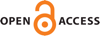Download this articleFor screen For printingRecent IssuesThe Journal About the Journal Editorial Board Editors’ Interests Subscriptions Submission Guidelines Submission Form Policies for Authors Ethics Statement ISSN: 1948-206X (e-only) ISSN: 2157-5045 (print) Author Index To Appear Other MSP JournalsMonge–Ampère gravitation as a $\Gamma$-limit of good rate functions

### Luigi Ambrosio, Aymeric Baradat and Yann Brenier

Vol. 16 (2023), No. 9, 2005–2040##### Abstract

Monge–Ampère gravitation is a modification of the classical Newtonian gravitation where the linear Poisson equation is replaced by the nonlinear Monge–Ampère equation. This paper is concerned with the rigorous derivation of Monge–Ampère gravitation for a finite number of particles from the stochastic model of a Brownian point cloud, following the formal ideas of a recent work by Brenier (Bull. Inst. Math. Acad. Sin. 11:1(2016), 23–41). This is done in two steps. First, we compute the good rate function corresponding to a large deviation problem related to the Brownian point cloud at fixed positive diffusivity. Second, we study the $\Gamma$-convergence of this good rate function, as the diffusivity tends to zero, toward a (nonsmooth) Lagrangian encoding the Monge–Ampère dynamic. Surprisingly, the singularities of the limiting Lagrangian correspond to dissipative phenomena. As an illustration, we show that they lead to sticky collisions in one space dimension.

##### Keywords
Monge–Ampère gravitation, large deviations, $\Gamma$-convergence, Lagrangian mechanics, interacting particle systems
##### Mathematical Subject Classification
Primary: 49J52, 60F10, 70F40, 70B05
##### Milestones